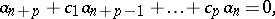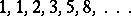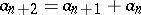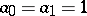# Recursive sequence

(diff) ← Older revision | Latest revision (diff) | Newer revision → (diff)

recurrent sequence

A sequencethat satisfies a relationwhereare constants. The relation permits one to compute the terms of the sequence one by one, in succession, if the firstterms are known. A classical example of such a sequence is the Fibonacci sequence(,). A recursive series is a power serieswhose coefficients form a recursive sequence. Such a series represents an everywhere-defined rational function.